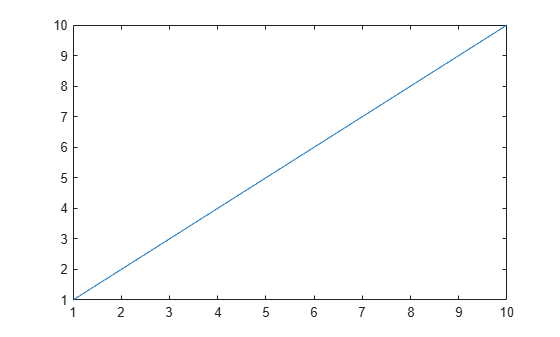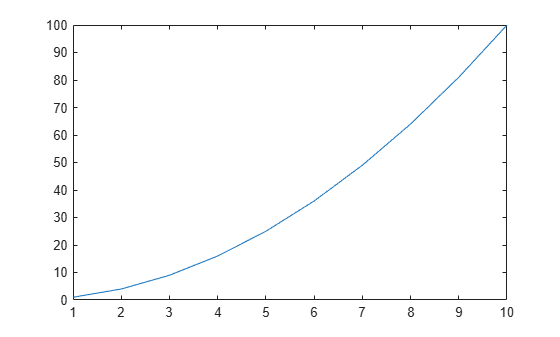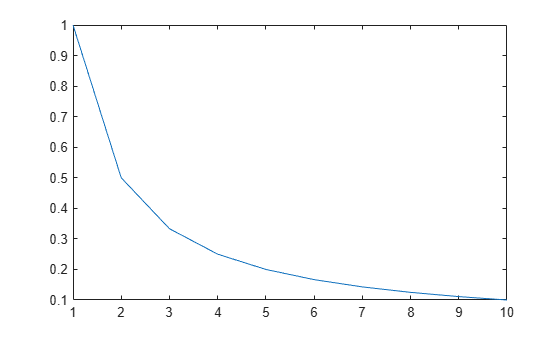Main Content

# close

Close one or more figures

## Syntax

``close``
``close(fig)``
``close all``
``close all hidden``
``close all force``
``status = close(___)``

## Description

example

````close` closes the current figure. Calling `close` is equivalent to calling `close(gcf)`.```

example

````close(fig)` closes the figure specified by `fig`.```

example

````close all` closes all figures whose handles are visible. A figure handle is hidden if the `HandleVisibility` property is set to `'callback'` or `'off'`.```

example

````close all hidden` closes all figures, including figures with hidden handles. ```

example

````close all force` closes all figures, including figures for which the `CloseRequestFcn` callback has been specified to prevent users from closing the figure window.```

example

````status = close(___)` returns the `status` of the close operation for any of the previous syntaxes. The function returns `1` if the figure or figures close and `0` otherwise. When specifying the output `status`, you must enclose input arguments that are character vectors in parentheses; for example, `status = close('all','hidden')`.```

## Examples

collapse all

Create two figures, each with a line plot.

```f1 = figure; plot(1:10)``````f2 = figure; plot((1:10).^2)```Close the first figure and display the value of `f1`.

```close(f1) f1```
```f1 = handle to deleted Figure ```

Close the current figure.

`close`

Create three figures, and then create a line plot. By default, the `plot` function targets the current figure (`f3`).

```f1 = figure; f2 = figure; f3 = figure; plot(1:10)```Close figures `f1` and `f2` simultaneously.

`close([f1 f2])`

Create two figures with specified numbers. Include a line plot in each figure.

```figure(1) plot(1:10)``````figure(2) plot((1:10).^2)```Close the second figure by passing its number to the `close` function.

`close(2)`

Create a figure, specify its name, and then create a line plot.

```figure('Name','Measured Data'); plot(1:10)```Close the figure using its name.

`close('Measured Data')`

Create two figures, each with a line plot.

```f1 = figure; plot(1:10)``````f2 = figure; plot((1:10).^2)```Close figure `f1`. Verify that the figure is closed by displaying its status.

`status = close(f1)`
```status = 1 ```

Create three figures whose handles are visible, and include a line plot in each figure.

```f1 = figure; plot(1:10)``````f2 = figure; plot((1:10).^2)``````f3 = figure; plot(1./(1:10))```Close all of the figures simultaneously.

`close all`

Create three figures, each with a line plot. Set the `HandleVisibility` property of the last figure to `'off'`.

```f1 = figure; plot(1:10)``````f2 = figure; plot((1:10).^2)``````f3 = figure('HandleVisibility','off'); plot(1./(1:10))```Close all of the figures. Notice that you cannot close `f3` by calling `close all` because it has a hidden handle.

`close all hidden`

The `CloseRequestFcn` property enables you to specify a close request callback, which executes whenever a user attempts to close the figure window. For example, you can display a dialog box asking to confirm or cancel the close operation or to prevent users from closing a figure that contains a UI.

Create a figure whose window cannot be closed by setting the `CloseRequestFcn` property to an empty character vector. Then, add a line plot to the figure.

```f1 = figure('CloseRequestFcn',''); plot(1:10)```Create a second figure with a line plot.

```f2 = figure; plot((1:10).^2)```If you try to close the figures using the `close all` syntax, MATLAB® closes only `f2`. To close both `f1` and `f2`, use the `close all force` syntax.

`close all force`

## Input Arguments

collapse all

Figure to close, specified as one or more `Figure` objects, figure numbers, or figure names.

• If `fig` is a figure number, MATLAB® searches for an existing figure in which the `Number` property is equal to `fig`. By default, the `Number` property value is displayed in the title of the figure.

• If `fig` is a figure name, MATLAB searches for an existing figure in which the `Name` property is equal to `fig`.

Example: `close(f)` closes the figure with handle `f`.

Example: `close([f1 f2])` closes the figures with handles `f1` and `f2`.

Example: `close(1)` closes the figure with number `1`.

Example: `close([1 2])` closes the figures with numbers `1` and `2`.

Example: `close('My Figure')` closes the figure with name `'My Figure'`.

Example: `close('My First Figure','My Second Figure')` closes the figures with names `'My First Figure'` and ```'My Second Figure'```.

## Tips

• To delete all figures unconditionally, use these statements:

```set(groot,'ShowHiddenHandles','on') c = get(groot,'Children'); delete(c)```

• When implementing a `CloseRequestFcn` callback, do not use a call to `close`. Calling `close` in the body of the callback sets up a recursion that results in a MATLAB warning. Instead, implement the callback using the `delete` function. `delete` removes the figure without executing the `CloseRequestFcn` callback.

• If you call `close` on a figure without specifying the `CloseRequestFcn` property, the default value of the property, `closereq`, unconditionally deletes the figure and closes its window. To prevent deletion when calling `close`, implement a `CloseRequestFcn` callback.

## Algorithms

The `close` function evaluates the `CloseRequestFcn` property of the specified figure `f` using this statement:

`eval(get(f,'CloseRequestFcn'))`

`CloseRequestFcn` enables you to either delay or abort the closing of a figure once `close` has been invoked. For example, you can display a dialog box to confirm that the user really wants to close the figure or save and clean up before closing.

The default value of `CloseRequestFcn`, `closereq`, closes the current figure using `delete(get(groot,'CurrentFigure'))`. If you specify an array of figure handles, `close` executes the callback specified by `CloseRequestFcn` for each figure.

If an error terminates the execution of a `CloseRequestFcn` callback, then the figure is not closed.

## See Also

### Properties

Introduced before R2006a

Download ebook